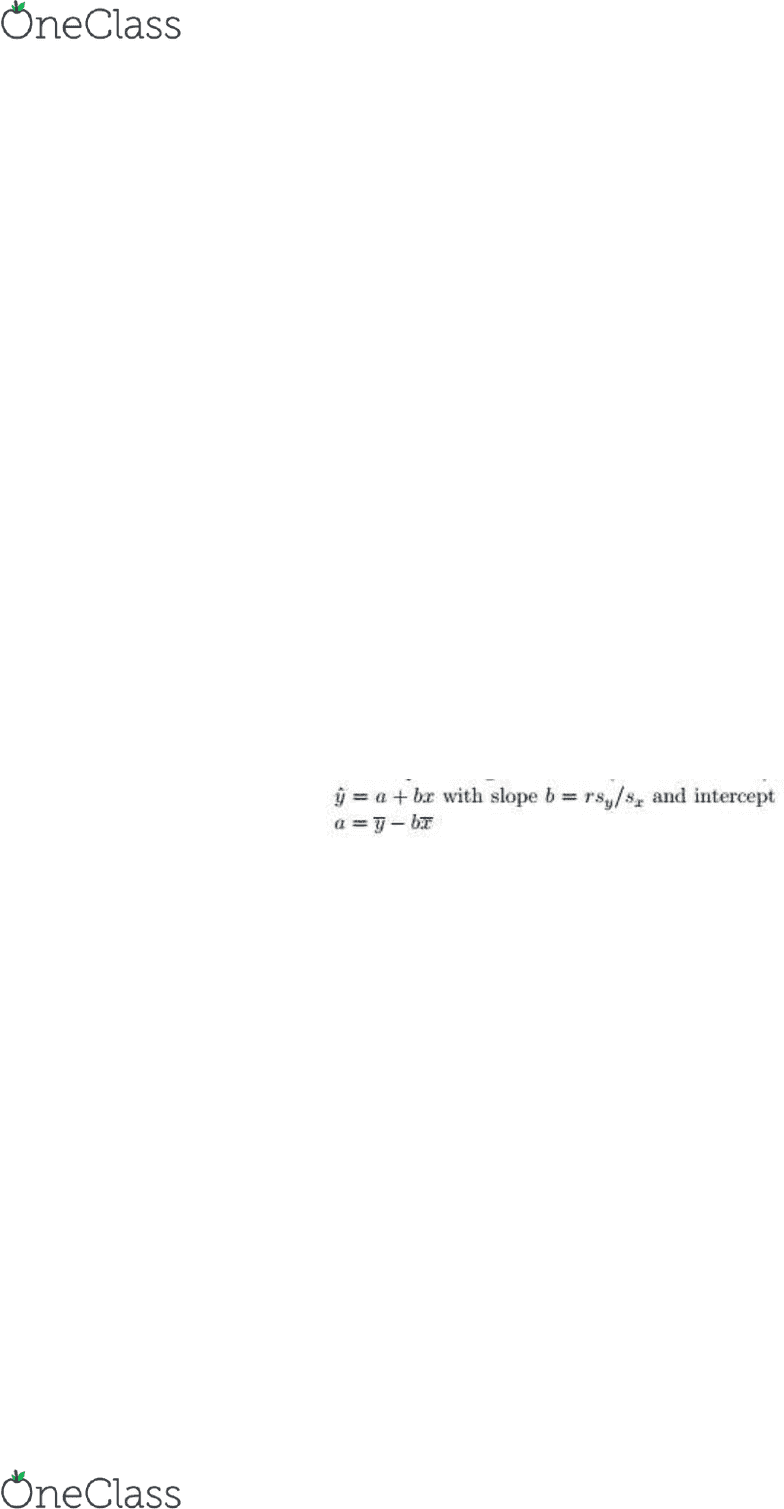Textbook Notes (270,000)
CA (160,000)
U of G (10,000)
SOAN (400)
Chapter 5

SOAN 3120 Chapter Notes - Chapter 5: Dependent And Independent Variables, Standard Deviation, Scatter Plot

Department
Sociology and Anthropology
Course Code
SOAN 3120
Professor
Andrew Hathaway
Chapter
5

This preview shows half of the first page. to view the full 3 pages of the document.Chapter 5 Regression
5.1 Regression Lines
- A regression line is a straight line that describes how a response variable y changes as an explanatory
variable x changes
o Often use regression lines to predict the value of y for a given value of x when we believe the
relationship between y and x is linear
- A straight line relating y to x has an equation of the form
y = a +bx
o In this equation, b is the slope, the amount by which y changes when x increases by one unit
o The number a is the intercept, the value of y when x = 0
- The slope of a regression line is an important numerical description of the relationship between the two
variables
o Although we need the value of the intercept to draw the line, this value is statistically meaningful
only when the explanatory variable can actually take values close to zero
5.2 The Least-Squares Regression Line
- In most cases, no line will pass exactly through all the points in a scatterplot
o Different people will draw different lines by eye, so we need a way to draw a regression line that
doesn’t depend on our guess of here the line should go
o Because we use the line to predict y from x, the prediction errors we make are errors in y, the
vertical direction in the scatterplot
- A good regression line makes the vertical distances of the points from the line as small as possible
- There are many ways to make the collection of vertical distances as small as possible; The most common
is the least-squares method
- The least-squares regression line of y on x is the line that makes the sum of the squares of the vertical
distance of the data points from the line as small as possible
- We write ŷ in the equation of the regression line to emphasize that the line gives a predicted response ŷ
for any x
o Because of the scatter of points about the line, the predicted response will usually not be exactly
the same as the actually observed response y
1. The distinction between explanatory and response variables is essential in regression
- Least-squares regression makes the distances of the data points form the line small only in the y direction
2. There is a close connection between correlation and the slope of the least-squares line
- The slope and correlation always have the same sign
- The formula for the slope b says more: along the regression line, a change of one standard deviation in x
corresponds to a change of r standard deviations in y
o When the variables are perfectly correlated, the change in the predicted response ŷ is the same
as the change in x
- As the correlation grows less strong, the prediction ŷ moves less in response to changes in x
3. The least-squares regression line always passed through the point (x-bar, y-bar) on the graph of y against x
4. The correlation r describes the strength of a straight-line relationship
- In the regression setting, this description takes a specific form: the square of the correlation, r2, is the
fraction of the variation in the values of y that is explained by the least-squares regression of y on x
find more resources at oneclass.com
find more resources at oneclass.com